# DFS algorithm

Traversal means visiting all the nodes of a graph. Depth first traversal or Depth first Search is a recursive algorithm for searching all the vertices of a graph or tree data structure. In this article, you will learn with the help of examples the DFS algorithm, DFS pseudocode and the code of the depth first search algorithm with implementation in C++, C, Java and Python programs.

## DFS algorithm

A standard DFS implementation puts each vertex of the graph into one of two categories:

1. Visited
2. Not Visited

The purpose of the algorithm is to mark each vertex as visited while avoiding cycles.

The DFS algorithm works as follows:

1. Start by putting any one of the graph's vertices on top of a stack.
2. Take the top item of the stack and add it to the visited list.
3. Create a list of that vertex's adjacent nodes. Add the ones which aren't in the visited list to the top of stack.
4. Keep repeating steps 2 and 3 until the stack is empty.

## DFS example

Let's see how the Depth First Search algorithm works with an example. We use an undirected graph with 5 vertices.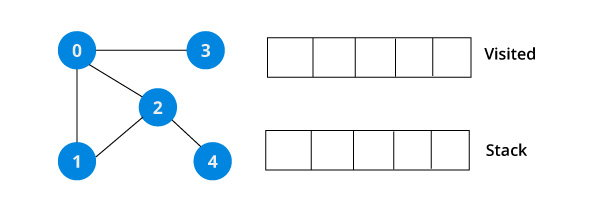We start from vertex 0, the DFS algorithm starts by putting it in the Visited list and putting all its adjacent vertices in the stack.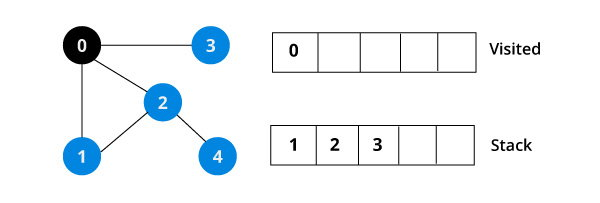Next, we visit the element at the top of stack i.e. 1 and go to its adjacent nodes. Since 0 has already been visited, we visit 2 instead.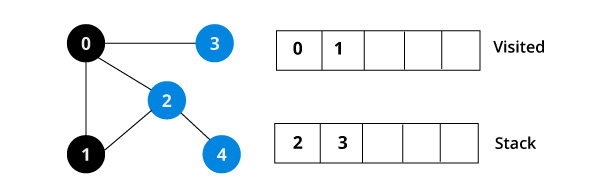Vertex 2 has an unvisited adjacent vertex in 4, so we add that to the top of the stack and visit it.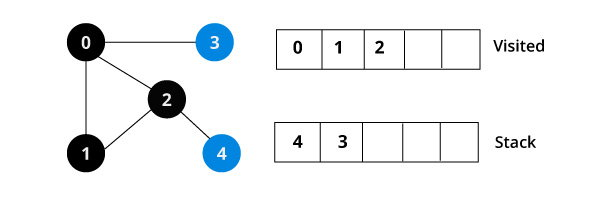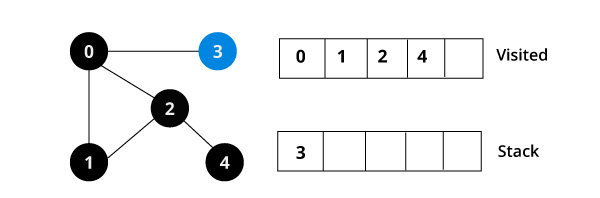After we visit the last element 3, it doesn't have any unvisited adjacent nodes, so we have completed the Depth First Traversal of the graph.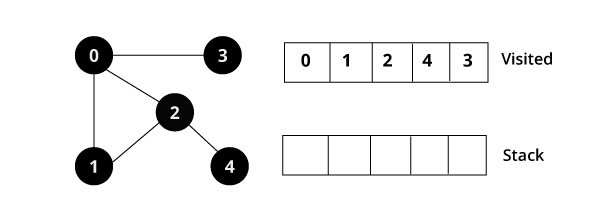## DFS pseudocode (recursive implementation)

The pseudocode for DFS is shown below. In the init() function, notice that we run the DFS function on every node. This is because the graph might have two different disconnected parts so to make sure that we cover every vertex, we can also run the DFS algorithm on every node.

``````DFS(G, u)
u.visited = true
if v.visited == false
DFS(G,v)

init() {
For each u ∈ G
u.visited = false
For each u ∈ G
DFS(G, u)
}``````

## DFS code

The code for the Depth First Search Algorithm with an example is shown below. The code has been simplified so that we can focus on the algorithm rather than other details.

### DFS in C

``````#include <stdio.h>
#include <stdlib.h>

struct node
{
int vertex;
struct node* next;
};

struct node* createNode(int v);

struct Graph
{
int numVertices;
int* visited;
struct node** adjLists; // we need int** to store a two dimensional array. Similary, we need struct node** to store an array of Linked lists
};

struct Graph* createGraph(int);
void printGraph(struct Graph*);
void DFS(struct Graph*, int);

int main()
{

struct Graph* graph = createGraph(4);

printGraph(graph);

DFS(graph, 2);

return 0;
}

void DFS(struct Graph* graph, int vertex) {

graph->visited[vertex] = 1;
printf("Visited %d \n", vertex);

while(temp!=NULL) {
int connectedVertex = temp->vertex;

if(graph->visited[connectedVertex] == 0) {
DFS(graph, connectedVertex);
}
temp = temp->next;
}
}

struct node* createNode(int v)
{
struct node* newNode = malloc(sizeof(struct node));
newNode->vertex = v;
newNode->next = NULL;
return newNode;
}

struct Graph* createGraph(int vertices)
{
struct Graph* graph = malloc(sizeof(struct Graph));
graph->numVertices = vertices;

graph->adjLists = malloc(vertices * sizeof(struct node*));

graph->visited = malloc(vertices * sizeof(int));

int i;
for (i = 0; i < vertices; i++) {
graph->visited[i] = 0;
}
return graph;
}

void addEdge(struct Graph* graph, int src, int dest)
{
// Add edge from src to dest
struct node* newNode = createNode(dest);

// Add edge from dest to src
newNode = createNode(src);
}

void printGraph(struct Graph* graph)
{
int v;
for (v = 0; v < graph->numVertices; v++)
{
printf("\n Adjacency list of vertex %d\n ", v);
while (temp)
{
printf("%d -> ", temp->vertex);
temp = temp->next;
}
printf("\n");
}
}``````

### Depth First Search in C++

``````#include <iostream>
#include <list>
using namespace std;

class Graph
{
int numVertices;
bool *visited;

public:
Graph(int V);
void DFS(int vertex);
};

Graph::Graph(int vertices)
{
numVertices = vertices;
visited = new bool[vertices];
}

{
}

void Graph::DFS(int vertex)
{

visited[vertex] = true;

cout << vertex << " ";

list::iterator i;
if(!visited[*i])
DFS(*i);
}

int main()
{
Graph g(4);

g.DFS(2);

return 0;
}``````

### DFS Java code

``````import java.io.*;
import java.util.*;

class Graph
{
private int numVertices;
private boolean visited[];

Graph(int vertices)
{
numVertices = vertices;
visited = new boolean[vertices];

for (int i = 0; i < vertices; i++)
}

{
}

void DFS(int vertex)
{
visited[vertex] = true;
System.out.print(vertex + " ");

while (ite.hasNext())
{
}
}

public static void main(String args[])
{
Graph g = new Graph(4);

System.out.println("Following is Depth First Traversal");

g.DFS(2);
}
}``````

### DFS in Python

``````def dfs(graph, start, visited=None):
if visited is None:
visited = set()